# MP Board Class 9th Science Solutions Chapter 8 Motion

## MP Board Class 9th Science Solutions Chapter 8 Motion

### Motion Intext Questions

Motion Intext Questions Page No. 100

Question 1.
An object has moved through a distance. Can it have zero displacement? If yes, support your answer with an example.
Yes, if an object start from a point and finally, reaches the same point. Then, it has distance but displacement is zero.Question 2.
A farmer moves along the boundary of a square field of side 10 m in 40 s. What will be the magnitude of displacement of the farmer at the end of 2 minutes 20 seconds?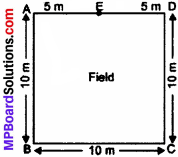Suppose farmer starts from A of the square field.
Now, he covered 10 m in 40 sec.
Now, 2 minutes 20 seconds =140 sec.
So, he cover in 140 seconds = $$\frac { 10 }{ 40 }$$ × 140 m
= $$\frac { 140 }{ 4 }$$m
= 35 m.
Now, in square field = 35m
AB + BC + CD + DE ($$\frac { 1 }{ 2 }$$AD)
So, he will reach at E.
Then, his displacement is AE = 5 m.

Question 3.
Which of the following is true for displacement?

1. It cannot be zero.
2. Its magnitude is greater than the distance travelled by the object.

1. False
2. False.

Motion Intext Questions Page No. 102

Question 1.
Distinguish between speed and velocity.

 Speed Velocity 1. it is the distance covered by a body per unit time. 2.  It is a scalar quantity. 1. It is the displacement covered by a body per unit time. 2.  It is a vector quantity.

Question 2.
Under what condition (s) is magnitude of average velocity of an object equal to its average speed?
When a body is moving in a straight line in a particular direction.

Question 3.
What does the odometer of an automobile measure?
It measures the distance travelled by the vehicle.Question 4.
What does the path of an object look like when it is in uniform motion?
Straight line.

Question 5.
During an experiment, a signal from a spaceship reached the ground station in five minutes. What was the distance of the spaceship from the ground station? The signal travels at the speed of light, that is 3 × 108 m/s.
Time taken by signal to reach ground station = 5 minutes
= 5 × 60 sec.
= 300 seconds.
Speed of the signal = 3 × 108 m/s.
∴ Distance between spaceship and ground station = speed × time
= 3 × 108 × 300
= 9 × 1010 m.

Motion Intext Questions Page No. 103

Question 1.
When will you say a body is in

1. Uniform acceleration?
2. Non – uniform acceleration?

1. A body is in uniform acceleration if its velocity changes uniformly with equal intervals of time. Example: Freely falling object.
2. A body is in non – uniform acceleration if its velocity changes non – uniformly with equal intervals of time. Example: A bus running in a city.

Question 2.
A bus decreases its speed from 80 km/h-1 to 60 km/h-1 in 5 s. Find the acceleration of the bus.
Initial velocity of the bus, u = 80 km/h
= $$\frac { 80\times 100 }{ 60\times 60 }$$ m/s
u = $$\frac { 800 }{ 36}$$ m/s
Final velocity of the bus, v = 60 km/h
= $$\frac { 60\times 100 }{ 60\times 60 }$$ m/s
v = $$\frac { 600 }{ 36 }$$ m/s
Time taken by bus to change its speeds, t = 5 seconds
∴ Acceleration, a = $$\frac { v-u }{ t }$$
= $$\frac { 600 }{ 36 }$$ – $$\frac { 800 }{ 36 }$$ ÷ 5
= $$\frac { 600-800 }{ 36 }$$ ÷ 5
= $$\frac { 200 }{ 36 }$$ × $$\frac { 1 }{ 5 }$$ m/s2
= $$\frac { -10 }{ 9 }$$ m/s2
a = -1.11 m/s2.Question 3.
A train starting from a railway station and moving with uniform acceleration attains a speed of 40 km/h-1 in 10 minutes. Find its acceleration.
Initial velocity, u = 0
Final velocity, v = 40 km/h
= $$\frac { 40\times 1000 }{ 60\times 60 }$$ m/s
= $$\frac { 400 }{ 36 }$$ m/s
= $$\frac { 200 }{ 18 }$$ m/s.
Time taken, t = 10 minutes
= 10 × 10 seconds = 100 seconds
a = $$\frac { v-u }{ t }$$
= $$\frac { 200 }{ 18 }$$ – 0 ÷ 100
= $$\frac { 200 }{ 18 }$$ ÷ 100
= $$\frac { 200 }{ 18 }$$ × $$\frac { 1 }{ 100 }$$ m/s2
= $$\frac { 1 }{ 9 }$$ m/s2
a = 0.018 m/s2.

Motion Intext Questions Page No. 107

Question 1.
What is the nature of the distance – time graphs for uniform and non – uniform motion of an object?
For uniform motion, it is a straight line.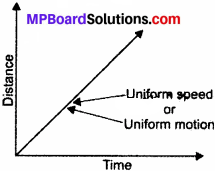For non – uniform motion, it is a curved line.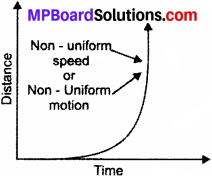Question 2.
What can you say about the motion of an object whose distance – time graph is a straight line parallel to the time axis?
It shows the body at rest.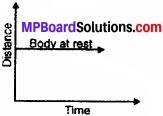Question 3.
What can you say about the motion of an object if its speed – time graph is a straight line parallel to the time axis?
It means body has constant speed or uniform motion with zero acceleration.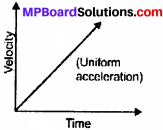Question 4.
What is the quantity which is measured by the area occupied below the velocity-time graph?
Distance travelled.

Motion Intext Questions Page No. 109 – 110

Question 1.
A bus starting from rest moves with a uniform acceleration of 0.1 m/s2 for 2 minutes. Find
(a) the speed acquired,
(b) the distance travelled.
Initial velocity, u = 0
Acceleration, a = 0.1 m/s2
Time, t = 2 min = 2 × 60 seconds
= 120 seconds
(a) Velocity, v = ?
We know, v = u + at
v = 0 + 0.1 × 120 m/s
= 12 m/s.

(b) Distance, s = ?
We Know, s = ut + $$\frac { 1 }{ 2 }$$ at2
= 0 × 120 + $$\frac { 1 }{ 2 }$$ × 0.1 × 120 × 120
= $$\frac { 1 }{ 2 }$$ × $$\frac { 0.1 }{ 10 }$$ × 120 × 120 m
= 720 m.

Question 2.
A train is travelling at a speed of 90 km/h-1. Brakes are applied so as to produce a uniform acceleration of – 0.5 m/s2. Find how far the train wilt go before it is brought to rest.
Initial velocity, u = 90 km/h
= $$\frac { 90\times 5 }{ 18 }$$ m/s
= 25 m/s
Final velocity, v = 0
Acceleration, a = -0.5 m/s2.
Distance, s = ?
We know,
v2 = u2 + 2as
02 = (25)2 + 2 × (-0.5) × s
0 = 625 – 1 × s
= 625 – 1 × s
= 625 – s
∴ s = 625 m.
∴ Distance travelled by the train = 625 m.

Question 3.
A trolley while going down an inclined plane, has an acceleration of 2 cm/s2. What will be its velocity in 3 s after the start?
Acceleration, a = 2 cm/s2
Time, t = 3 sec.
Initial velocity, u = 0
Final velocity, v = ?
Now, v= u + at
v = 0 + 2 × 3
= 6 cm/s.Question 4.
A racing car has a uniform acceleration of 4 m/s2. What distance will it cover in 10 s after start?
Acceleration, a = 4 m/s2
Time, t = 10 sec,
Initial velocity, u = 0
Distance, s = ?
Now, s = ut + $$\frac { 1 }{ 2 }$$ at2
s = 0 × 10 + $$\frac { 1 }{ 2 }$$ × 4 × (10)2
s = $$\frac { 1 }{ 2 }$$ × 4 × 100 m
s = 200 m.
So, the distance covered is 200 m.

Question 5.
A stone is thrown in a vertically upward direction with a velocity of 5 m/s-1. If the acceleration of the stone during its motion is 10 m/s-2 in the downward direction, what will be the height attained by the stone and how much time will it take to reach there?
Initial velocity, u = 5 m/s
Final velocity, v = 0
Acceleration, a = -10 m/s2 (∴ It is from opposite direction)
Height attained, s = ?
Time taken, t = ?
Now, V2 = u2 + 2 as
(0)2 = (5)2 + 2 × (-10) × s
0 = 25 – 20s
⇒ 20s = 25
∴ s = $$\frac { 25 }{ 20 }$$ = $$\frac { 5 }{ 4 }$$ = 1025m
Now, v = u + at
0 = 5 + (-10) × t
0 = 5 – 10 × t
-5 = -10 × t
∴ t = $$\frac { -5 }{ -10 }$$ = $$\frac { 1 }{ 2 }$$ = 0.5 sec
So, height attained by the stone is 1.25 m and time taken to reach there is 0.5 sec.

### Motion NCERT Textbook Exercises

Question 1.
An athlete completes one round of a circular track of diameter 200 m in 40 s. What will be the distance covered and the displacement at the end of 2 minutes 20 s?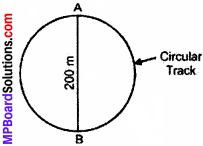Diameter of the track = 200 m
So, radius = $$\frac { 200 }{ 2 }$$ m
= 100m
Circumference = 2πr
= 2 × $$\frac { 22 }{ 7 }$$ × 100 m
= $$\frac { 4400 }{ 7 }$$ m.
Now, athlete covers 200 m in 40 s.
So, rounds covered in 40 seconds = 1
Rounds covered in 1 sec = $$\frac { 1 }{ 40 }$$
Rounds covered in 140 sec = $$\frac { 1 }{ 40 }$$ × 140
= 3.5 rounds
So, distance covered = 3.5 × circumference
= $$\frac { 3.5 }{ 10 }$$ × $$\frac { 4400 }{ 7 }$$ m
= 2200 m.
And, displacement after 3.5 rounds
= Diameter of track
= 200 m.

Question 2.
Joseph jogs from one end A to the other end B of a straight 300 m road in 2 minutes 30 seconds and then turns around and jogs 100 m back to point C in another 1 minute. What are Joseph’s average speeds and velocities in jogging (a) from A to B and (b) from A to C?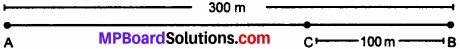(a) A to B,
Time taken, t = 2 min, 30 sec
= 150 sec.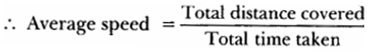= $$\frac { 300 }{ 150 }$$ m/s = 2 m/s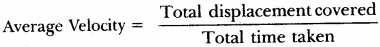= $$\frac { 300 }{ 150 }$$ m/s = 2 m/s.

(b) A to C,
Time taken = Time from A to B + Time from B to C
= 2 min 30 sec + 1 min
= 150 sec + 60 sec
= 210 sec.
Total distance covered (AB + BC) = (300 + 100) m
= 400 m
Displacement = AC = (300 – 100) m
= 200 m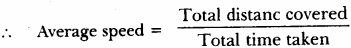= $$\frac { 400 }{ 210 }$$ m/s = 1.9 m/s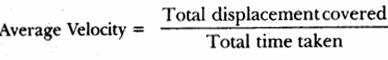= $$\frac { 200 }{ 210 }$$ m/s = 0.95 m/s.

Question 3.
Abdul, while driving to school, computes the average speed for his trip to be 20 kmh-1. On his return trip along the same route, there is less traffic and the average speed is 30 kmh-1. What is the average speed for Abdul’s trip?
Let the distance between Abdul’s home and school = x km.
Lets time taken from home to school = t1 hr.
and time taken from school to home = t2 hr.
Now,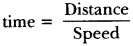∴ t1 = $$\frac { x }{ 20 }$$ hr.
t2 = $$\frac { x }{ 30 }$$ hr.
Now,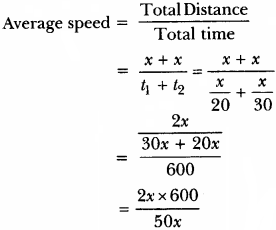∴ Average Speed = $$\frac { 120 }{ 5 }$$ 24 km/h.

Question 4.
A motorboat starting from rest on a lake accelerates in a straight line at a constant rate of 3.0 ms-2 for 8.0 s. How far does the boat travel during this time?
Initial velocity, u = 0
Acceleration, a = 3.0 m/s2
= 3 m/s2
Time, t = 8 sec
Now,
s = ut + $$\frac { 1 }{ 2 }$$ at2
s = 0 × 8 + $$\frac { 1 }{ 2 }$$ × 3 × (8)2
= 0 + $$\frac { 1 }{ 2 }$$ × 3 × 64
s = 96 m.
∴ Distance Travelled by Boat is 96 m.

Question 5.
A driver of a car travelling at 52 kmh-1 applies the brakes and accelerates uniformly in the opposite direction. The car stops in 5 s. Another driver going at 34 kmh-1 in another car applies his brakes slowly and stops in 10 s. On the same graph paper, plot the speed versus time graphs for the two cars. Which of the two cars travelled farther after the brakes were applied?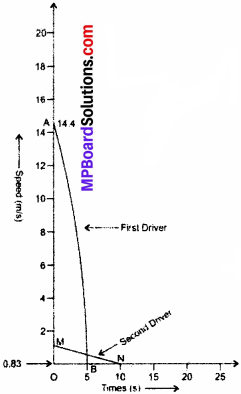We have,
Speed of first driver = 52 km/h
= $$\frac { 52\times 1000 }{ 60\times 60 }$$ m/s = 14.4 m/s
Speed of second driver = 3 km/h
= $$\frac { 3\times 1000 }{ 60\times 60 }$$ m/s = 0.83 m/s
Now, distance covered by first driver = Area of ΔAOB
= $$\frac { 1 }{ 2 }$$ × OA × OB
= $$\frac { 1 }{ 2 }$$ × 14.4 × 5 = 36.1 m
Distance covered by second driver = Area of ΔMON
= $$\frac { 1 }{ 2 }$$ × OM × ON
= $$\frac { 1 }{ 2 }$$ × O.83 × 10 = 4.16 m
So, first driver covers more distance.

Question 6.
Figure below shows the distance-time graph of three objects A, B and C. Study the graph and answer the following questions: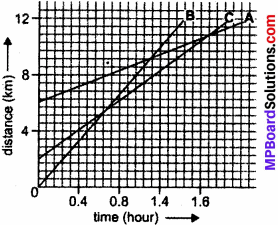1. Which of the three is travelling the fastest?
2. Are all three ever at the same point on the road?
3. How far has C travelled when B passes A?
4. How far has B travelled by the time it passes C?

1. B is travelling the fastest.
2. No.
3. C was at around 9 km from origin when B passes A.
4. B travelled around 5.5 km when it passes C.Question 7.
A ball is gently dropped from a height of 20 m. If its velocity increases uniformly at the rate of 10 ms-2, with what velocity will it strike the ground? After what time will it strike the ground?
Distance, s = 20 m
Initial velocity, u = 0
Acceleration, a = 10 m/s2
Now, s = ut +$$\frac { 1 }{ 2 }$$ at2
20 = 0 × t + $$\frac { 1 }{ 2 }$$ × 10 × t2
20 = 5 × t2
∴ t2 = $$\frac { 20 }{ 5 }$$ = 4
∴ t = 2 sec.
Also, v = u + at
v = 0 + 10 × 2
y = 20 m/s.
So, it will strike the ground after 2 seconds with the velocity of 20 m/s.

Question 8.
The speed – time graph for a car is shown in figure below: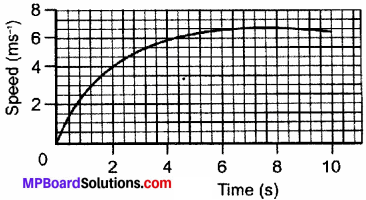(a) Find how far does the car travel in the first 4 seconds. Shade the area on the graph that represents the distance travelled by the car during the period.
(b) Which part of the graph represents uniform motion of the car?
(a) The distance travelled by car in first 4 seconds
= Area of triangular like region OAB
= $$\frac { 1 }{ 2 }$$ × AB × OB = $$\frac { 1 }{ 2 }$$ × 6.9 × 4
= 12 m (approximately).

(b) Part of the graph after 5.5 seconds shows the uniform motion.

Question 9.
State which of the following situations are possible and give an example for each of these:

1. an object with a constant acceleration but with zero velocity.
2. an object moving in a certain direction with an acceleration in the perpendicular direction. Both are possible.

1. Freely falling.
2. Motion of an object in a circular path.

Question 10.
An artificial, satellite is moving in a circular orbit of radius 42250 km. Calculate its speed if it takes 24 hours to revolve around the earth.
Radius of the orbit = 42250 km = 42250 × 1000 m.
Time taken to complete one revolution = 24 hours.
= 24 × 60 × 60 sec
Distance covered by satellite to complete 1 revolution = 2πr
= 2 × $$\frac { 22 }{ 7 }$$ × 42250 × 1000 m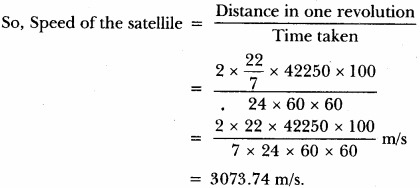Motion Multiple Choice Questions

Question 1.
Deceleration of a body is expressed in _______ .
(a) m
(b) ms-1
(c) ms-2
(d) -ms-2
(c) ms-2.

Question 2. A car goes from town A to another town B with a speed of 40 km/h and returns back to the town A with a speed of 60 km/h. The average speed of the car during the complete journey is _______ .
(a) 48 km/h
(b) 50 km/h
(c) Zero
(d) None of these.
(a) 48 km/h

Question 3.
A ball is thrown vertically upwards. It rises to a height of 50 m and comes back to the thrower, _______ .
(a) The total distance covered by the ball is zero.
(b) The net displacement of the ball is zero.
(c) The displacement is 100 m.
(d) None of the above.
(b) The net displacement of the ball is zero.

Question 4.
The SI unit of velocity is _______ .
(a) ms-1
(b) ms-2
(c) ms-3
(d) Nm-1.
(a) ms-1

Question 5.
In 12 minutes a car whose speed is 35 km/h travels a distance of _______ .
(a) 7 km
(b) 3.5 km
(c) 14 km
(d) 28 km.
(a) 7 km

Question 6.
A 50 m long train passes over a 250 m long bridge at a velocity of 60 km/h. How long will it take to pass completely over the bridge?
(a) 18 s
(b) 20 s
(c) 24 s
(d) none of these.
(a) 18 sQuestion 7.
The initial velocity of a train which is stopped in 20 s by applying brakes (retardation due to brakes being 1.5 ms-2) is _______ .
(a) 30 ms-1
(b) 30 cm-1
(c) 20 cm-1
(d) 24 ms-1.
(a) 30 ms-1

Question 8.
A wooden slab starting from rest, slides down a 10 m long inclined plane with an acceleration of 5 ms2. What would be its speed at the bottom of the inclined plane?
(a) 10 ms-1
(b) 12 ms-1
(c) 10 cm s-1
(d) 12 cm s-1.
(a) 10 ms-1

Question 9.
m/s2 is the SI unit of _______ .
(a) Distance
(b) Displacement
(c) Velocity
(d) Acceleration.
(d) Acceleration.

Question 10.
A car increases its speed from 20 km/h to 50 km/h in 10 seconds. Its acceleration is _______ .
(a) 30 m/s2
(b) 3 m/s2
(c) 18 m/s2
(d) 0.83 m/s2.
(d) 0.83 m/s2.

Motion Very Short Answer type Questions

Question 1.
Define uniform motion.
When an object covers equal distances in equal intervals of time: it is said to be in uniform motion.

Question 2.
Define speed.
It is defined as the distance travelled by an object in unit time. Its unit is m/s.

Question 3.
Define average speed.
The total distance travelled by an object divided by the total time taken.

Question 4.
Define velocity.
Velocity is the speed of an object moving in definite direction.

Question 5.
Define acceleration.
Change in the velocity of an object per unit time.
a = $$\frac { v-u }{ t }$$, S.I. unit is m/s2.

Question 1.
While plotting a distance – time graph, why do we plot time on x – axis.
While plotting a distance – time graph, we should plot time on x – axis as, all independent quantities are plotted on x – axis.

Question 2.
What is odometer?
Odometer is a device fitted in the automobiles to show the distance travelled.

Question 3.
Draw a distance – time graph that represents uniform speed.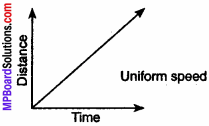Question 4.
With the help of distance – time graph show that the object is stationary.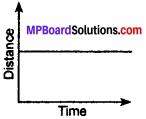Question 5.
What does the area under velocity – time graph represent?
The area under the velocity – time graph represents the displacement.

Question 6.
A body travels a distance from A to B, its physical quantity is measured to be -15 m/s. Is it speed or velocity? Give reason for the answer.
The physical quantity is -15 m/s. It represents velocity because sign of speed cannot be a negative. Negative sign indicates the opposite direction.

Question 7.
What type of velocity – time graph will you get for a particle moving with a constant acceleration?
For a particle moving with a constant acceleration velocity – time graph will be a straight line inclined to x – axis.

Question 8.
Define uniform circular motion.
When a body moves in a circular path with uniform speed, its motion is called uniform circular motion.

Question 9.
What is deceleration?
When the speed of an object decreases, the object is said to be decelerating. In this case, the direction of acceleration is opposite to the velocity of the object.

Question 10.
What conclusion do you draw about acceleration of the particle in motion from given velocity – time graphs?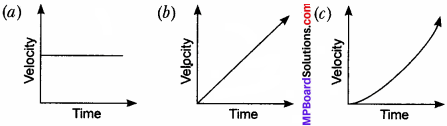(a) No acceleration.
(b) Uniform acceleration.
(c) Non – uniform acceleration.

Question 11.
With the help of graph, show the uniform acceleration and uniform retardation of a body.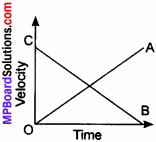• OA – Shows uniform acceleration.
• CB – Shows uniform retardation.

Question 12.
Give difference between acceleration and velocity.
table

Question 1.
How can we get speed from distance – time graph?
Let us assume that an object moves with a uniform speed. The distance – time graph will be a straight line.
Let d1 be the distance covered in time t1.
Let d2 be the distance covered in time t2.
∴ d2 – d1 will be the distance covered in t2 – t2.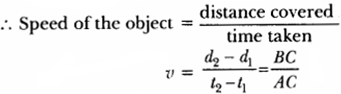But, here $$\frac { BC }{ AC }$$ = slope of the graph.
Conclusion:
To find the speed from distance – time graph, we can get its slope which is equal to the speed of the object.

Question 2.
Represent a graph that shows the following:
(a) Uniform speed
(b) Non – uniform speed
(c) Stationary object.
Time is taken on x – axis as it is independent quantity and all dependent quantities are taken along y – axis.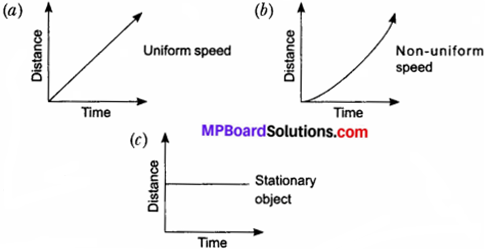Question 3.
(a) What is motion?
(b) State types of motion.
(c) Derive the unit for acceleration.
(a) Motion: An object is said to be in motion when its position changes with time. We describe the location of an object by specifying a reference point. Motion is relative. The total path covered by an object is said to be the distance travelled by it.

(b) There are two types of motion: Uniform motion and non – uniform motion

• Uniform motion: When an object covers equal distances in equal intervals of time, it is said to be in uniform motion.
• Non – uniform motion: Motion where object cover unequal distances in equal intervals of time.

(c) Acceleration, ‘a’ is change in velocity per unit time.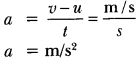Question 4.
(a) What is uniform circular motion?
(b) An athlete runs on a circular track, whose radius is 50 m with a constant speed. It takes 50 seconds to reach point B from starting point A. Find:

1. the distance covered.
2. the displacement.
3. the speed.

(a) When a body moves in a circular path with uniform speed, its motion is called uniform circular motion.

Time = 50 s
The distance covered by an athlete A to B i.e., semicircle of the track
∴ Circumference = 2πr
circumference half of = πr.

Question 5.
Derive the equation for velocity – time relation (v = u + at) by graphical method.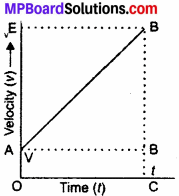Let, the initial velocity of a body be, u = OA = CD.
The final velocity of a body be, v = OE = CB
Time, t = OC = AD
BC = BD + DC
= BD + OA
v = BD + u
∴ BD =v – u …….. (1)
In velocity – time graph, slope gives acceleration.
∴ a = $$\frac { BD }{ AD }$$ = $$\frac { BD }{ OC }$$ = OC = t
we get, a = $$\frac { BD }{ t }$$
∴ BD = at …….. (2).

Question 6.
A car accelerates uniformly from 20 km/h to 35 km/h in 5 s. Calculate (i) the acceleration and (ii) the distance covered by the car in that time.
u =20 km/h = 5.5 m/s
v =35 km/h = 9.72 m/s
t = 5 s
a = $$\frac { v-u }{ t }$$
= $$\frac { 9.7-5.5 }{ 5 }$$
a = $$\frac { 4.2 }{ 5 }$$
= 0.84 m/s2.

(ii) The distance covered by the car s = ut + $$\frac { 1 }{ 2 }$$ at2
= 5.5 × 5 + $$\frac { 1 }{ 2 }$$(0.84)(5)2
= 27.5 + $$\frac { 1 }{ 2 }$$ × 0.84 × 25
= 27.5 + 10.5
s = 38 m.

Question 7.
Draw graph to show the following:
(a) Uniform acceleration.
(b) Non – uniform acceleration.
(c) Uniform motion.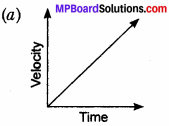Uniform acceleration – A body travels equal distances in equal intervals of time.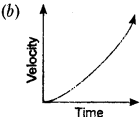Non – uniform acceleration – A body travels unequal distances in equal intervals of time.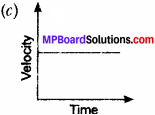Uniform motion or zero acceleration A body moves with constant speed in same line.

Question 8.
How can you get the distance travelled by an object from its speed – time graph?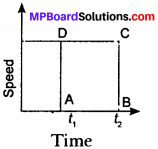Suppose an object is moving with a same speed v, the distance covered by this object when it was at (point A) t1 to t2 is:
AB = t2 – t1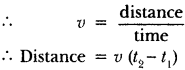∴ s = Area of rectangle ABCD
∴ To find the distance travelled by a body from its speed – time graph, we need to find the area enclosed by the graph.

Motion Higher Order Thinking Skills (HOTS)

Question 1.
A blacksmith strikes a nail with a hammer of mass 500 g moving with a velocity of 20 ms-1. The hammer comes to rest in 0.02 s after striking the nail. Calculate the force exerted by the nail on the hammer.
m = 500 g = $$\frac { 1 }{ 2 }$$ kg
u = 20 ms-1 , v = 0
t = 0.02 s, F = ?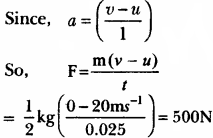Therefore, force exerted by nail on the hammer = 500 N.

Motion Value Based Question

Question 1.
A jeep was moving at a speed of 120 km/h on an highway. All of a sudden tyre of the jeep got punctured and burst The driver of the jeep did not apply sudden brakes but he slowed down its speed and finally stopped it at the road side. All the passengers in the jeep were shocked but happy with the driver’s decison.

1. What would have happened if the driver applied sudden brakes?
2. On applying brakes in which direction would the passengers fall?
3. What value of driver is reflected in this act?